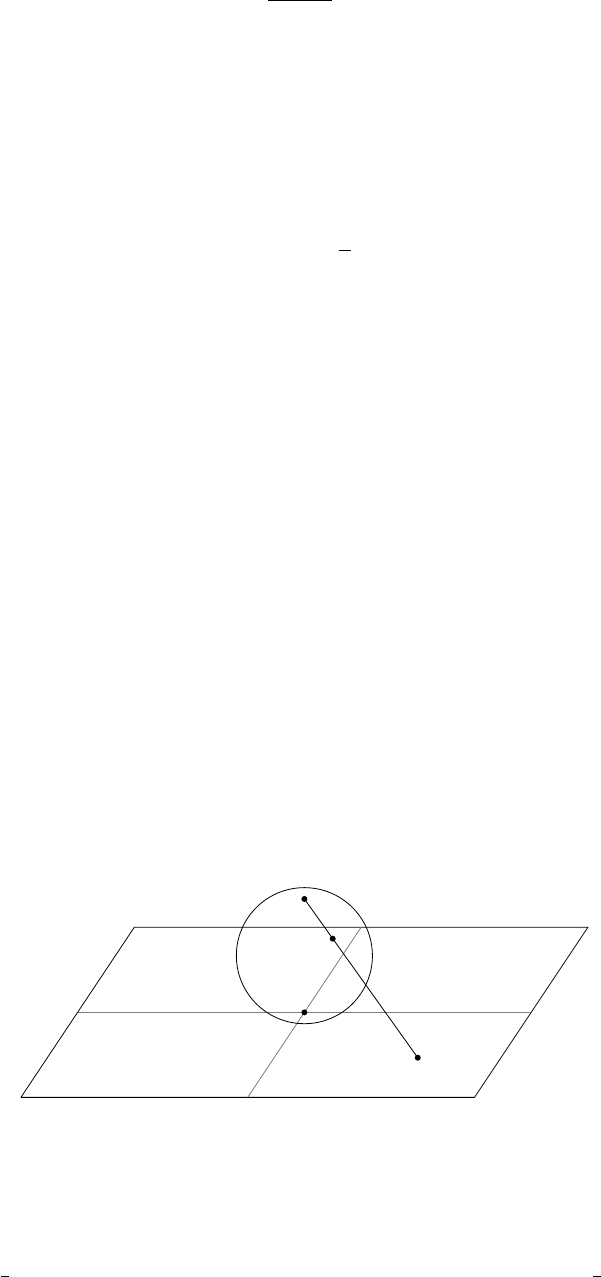1Analytic functions

IB Complex Methods1.1 The complex plane and the Riemann sphere
We begin with a review of complex numbers. Any complex number
z C
can
be written in the form
x
+
iy
, where
x
=
Re z
,
y
=
Im z
are real numbers. We
can also write it as re
, where
Definition
(Modulus and argument)
.
The modulus and argument of a complex
number z = x + iy are given by
r = |z| =
p
x
2
+ y
2
, θ = arg z,
where x = r cos θ, y = r sin θ.
The argument is defined only up to multiples of 2
π
. So we define the following:
Definition
(Principal value of argument)
.
The principal value of the argument
is the value of θ in the range (π, π].
We might be tempted to write down the formula
θ = tan
1
y
x
,
but this does not always give the right answer it is correct only if
x >
0. If
x 0, then it might be out by ±π (e.g. consider z = 1 + i and z = 1 i).
Definition
(Open set)
.
An open set
D
is one which does not include its boundary.
More technically,
D C
is open if for all
z
0
D
, there is some
δ >
0 such that
the disc |z z
0
| < δ is contained in D.
Definition
(Neighbourhood)
.
A neighbourhood of a point
z C
is an open set
containing z.
The extended complex plane
Often, the complex plane
C
itself is not enough. We want to consider the point
as well. This forms the extended complex plane.
Definition
(The extended complex plane)
.
The extended complex plane is
C
=
C {∞}
. We can reach the “point at infinity” by going off in any direction
in the plane, and all are equivalent. In particular, there is no concept of
−∞
.
All infinities are the same. Operations with are done in the obvious way.
Sometimes, we do write down things like
−∞
. This does not refer to a
different point. Instead, this indicates a limiting process. We mean we are
approaching this infinity from the direction of the negative real axis. However,
we still end up in the same place.
Conceptually, we can visualize this using the Riemann sphere, which is a
sphere resting on the complex plane with its “South Pole” S at z = 0.
S
N
P
z
For any point
z C
, drawing a line through the “North Pole”
N
of the sphere
to
z
, and noting where this intersects the sphere. This specifies an equivalent
point
P
on the sphere. Then
is equivalent to the North Pole of the sphere
itself. So the extended complex plane is mapped bijectively to the sphere.
This is a useful way to visualize things, but is not as useful when we actually
want to do computations. To investigate properties of
, we use the substitution
ζ
=
1
z
. A function
f
(
z
) is said to have a particular property at
if
f
(
1
ζ
) has
that same property at
ζ
= 0. This vague notion will be made precise when we
have specific examples to play with.